### What is Half wave Rectifier?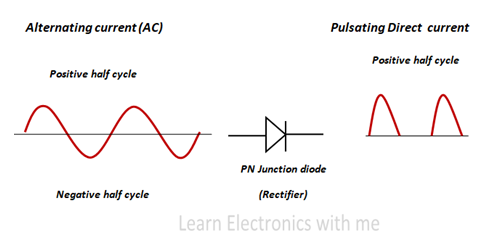Halfwave Rectifier

Half wave rectifier is a type of rectifier which converts the positive half cycle of the input alternating current (AC) into pulsating direct output current (DC) . It allows only the positive half cycle and blocks the negative cycle.

### Half wave Rectifier circuit: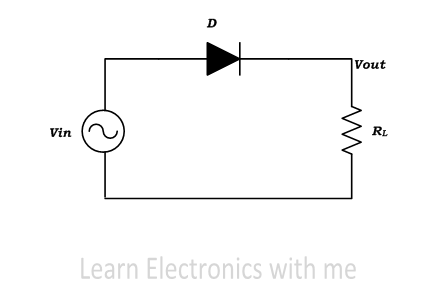Halfwave Rectifier circuit

The basic halfwave rectifier circuit consists of three main components, they are Alternating current input source, one diode and resistive load.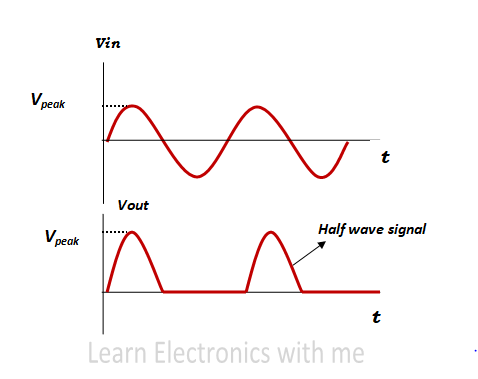Input and output current of Halfwave Rectifier

When the input alternating current is applied and when the diode is forward biased it allows the current to pass through the diode. It acts like closed circuit.

When the diode is reverse biased, the diode does not allows the current to flow. It acts like short circuit. Thus the output voltage consists of only positive half cycle and no negative half cycle.

### Half wave Rectifier with Capacitor filter (Soothing Capacitor):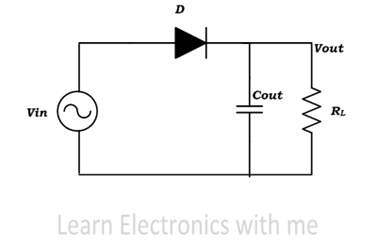Half wave Rectifier with Capacitor Filter

The output which we receive from half wave rectifier is a pulsating direct current that increases to maximum and decreases to zero. But we need a smooth direct current without variations or ripples.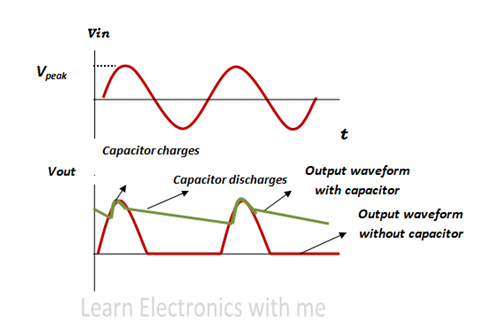Output current of the Halfwave rectifier with filter

When the input alternating current is applied and when forward biased the capacitor starts charging and when the output current reaches the maximum the capacitor voltage is also at maximum, when the output current reduces and reaches zero the capacitor starts discharging.

### Limitation:

Halfwave rectifier has some limitation, if the load resistance is of small value the capacitor discharges very quickly so the ripples increases. So Halfwave rectifier is used in low power application.

### Halfwave Rectifier Calculations: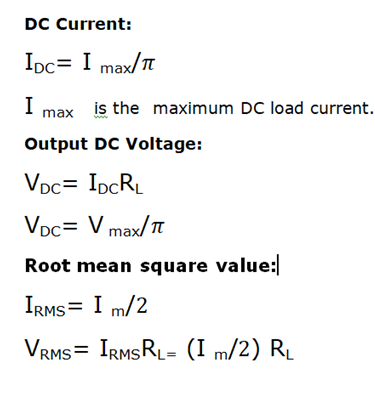Half wave Rectifier Calculations